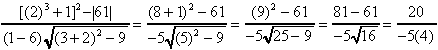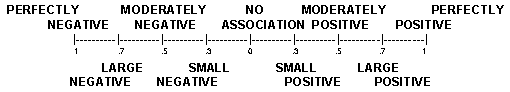previous lesson

Gamma

ORDINAL DATA: AssociationGamma Measure of Association(ordinal data):Where: fa = frequency of agreements in rank order
fi = frequency of inversions in rank order.

A. Procedure for data without ties:

1. Put one variable in order from largest to smallest (or highest to lowest).
2. Rearrange other variables to correspond.
3. Count fa and fi on second variable:
a) fa for each score is the number of scores located above it which are larger than it is. Sum these.
b) fi for each score is the number of scores located above it which are smaller than it is. Sum these.
Example:

Q: What is the relationship between I.Q. and income?
A: Rearrange one of the variables from largest to smallest (choose I.Q.), then rearrange the other variable.

 Person I.Q. Income 001 138 950 002 101 750 003 108 600 004 115 800 005 140 1000

transform to a table in order of one variable (highest to lowest here)

 Person I.Q. Income fa fi E 140 1000 0 0 = (9-1)/(9+1) Q 138 950 1 0 = D 115 800 2 0 8/10 = +.80 C 108 600 3 0 B 101 750 3 1 fa = 9 1=fi

Interpretation:Use above scale or Convert to a percent and include in the statements, "There is a ________ association between (variable 1) and (variable 2)." for a positive relationship - "There is ____% more agreement than disagreement in the rank order of (variable 1) and (variable 2)." For a negative relationship - "There is _____% more disagreement than agreement in the rank order of (variable 1) and (variable 2)."

Example: G = +.80 = large positive association between I.Q. and income. 80% more agreement than disagreement in the rank order of I.Q. and income.

B. Procedure for data with ties:
1. Put data in a table (in standard form).
2. To get fa, multiply the number in each cell by the sum of the numbers in the cells located both below and to the right of it (the numbers not in the same row or column). Start in the left upper corner and work across the first row. Then go to the next row and start at the left side working again across the row. Sum these products.
3. To get fi, multiply the number in each cell by the sum of the numbers in the cells located both below and to the left of it. Continue as with fa but start with the right upper corner and work to the left. Sum these products.

Example:

# OF CLASS ABSENCES

 HI MED LO HI 9 26 13 SUNNY DAYS MED 19 75 83 LOW 16 56 110

Q: What is the association between sunny days and class absences?
A: Notice there are many ties in the data, but only one tie is needed to qualify for procedure for data with ties. The data is already in a (standard form) table.

# OF CLASS ABSENCES

 HI MED LO HI 9 26 13 SUNNY DAYS MED 19 75 83 LOW 16 56 110

fa = below and to the right = 9(75 + 83 + 56 + 110)+26(83 + 110)+13(0) +19(56 + 110)+75(110)+83(0) +16(0)+56(0)+ 110(0) = 19,338

* Right hand column and bottom row cells both yield zeros so it is not necessary to include them

# OF CLASS ABSENCES

 HI MED LO HI 9 26 13 SUNNY DAYS MED 19 75 83 LOW 16 56 110

fi = below and to the left = 13(19 + 75 + 16 + 56) + 26(19 + 16) +9(0+83(16 + 56) +75(16) +19(0) + 110(0) +56(0)+ 16(0) = 10,244

*The left hand column and bottom row cells both yield zeros so it is not necessary to include them.

G = 19338 - 10244 = .31 Interpretation: Moderately small positive relationship and 31%
19338 + 10244 more agreement than disagreement in rank order of sunny days and class absences.